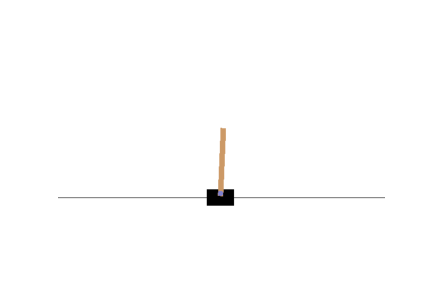# Training the Neural Network

• From the previous section, it is pretty clear that the cartpole is quite unstable and wobbly. Let us now head towards training the neural network with our basic policy.

• As discussed already, we shall continue with our basic policy and choose a random threshold.

• We can make the same net play in 50 different environments in parallel (this will give us a diverse training batch at each step), and train for 5000 iterations. We also reset environments when they are done. We train the model using a custom training loop so we can easily use the predictions at each training step to advance the environments.

INSTRUCTIONS
• Set `np` seed to 42:

``````np.random.seed(42)
``````
• Set the number of environments `n_environments` to `50`.

``````n_environments = 50
``````
• Set the number of iterations to `5000`.

``````n_iterations = 5000
``````
• Initialize 50 different cartpole environments:

``````envs = [gym.make("CartPole-v1") for _ in range(n_environments)]
``````
• Set different seeds to each environment with their respective indices as per the above list.

``````for index, env in enumerate(envs):
env.seed(index)
``````
• Reset all the environments and get the `observations` for all the environment:

``````observations = [env.reset() for env in envs]
``````
• Initialize the `optimizer` to `keras.optimizers.RMSprop()`:

``````optimizer = << your code comes here >>
``````
• Initialize the `loss` function to be `keras.losses.binary_crossentropy`:

``````loss_fn =  << your code comes here >>
``````
• Now for each iteration, let us set the target probabilities of the action. If angle < 0, we want proba(left) = 1., or else proba(left) = 0. Then, we shall fit the observations to the model and calculate the gradients based on the loss with respect to the targets. We shall use the probabilities predicted by the model and determined the `actions` and proceed for the next step, and the iterations continue.

``````for iteration in range(n_iterations):
# if angle < 0, we want proba(left) = 1., or else proba(left) = 0.
target_probas = np.array([([1.] if obs < 0 else [0.])
for obs in observations])

left_probas = model(np.array(observations))
loss = tf.reduce_mean(loss_fn(target_probas, left_probas))
print("\rIteration: {}, Loss: {:.3f}".format(iteration, loss.numpy()), end="")

actions = (np.random.rand(n_environments, 1) > left_probas.numpy()).astype(np.int32)
for env_index, env in enumerate(envs):
obs, reward, done, info = env.step(actions[env_index])
observations[env_index] = obs if not done else env.reset()
``````
• Let us visualize the cartpole after training:This seems to have learned the policy better. Now, we shall work towards making the pole lesser wobbly. One way to do this might be by allowing the cartpole to learn/explore for itself a better policy. So rather than we decide on the policy, we shall now modify the algorithm such that the network itself learns a better policy. Let's see how!

No hints are availble for this assesment

Answer is not availble for this assesment

Note - Having trouble with the assessment engine? Follow the steps listed here